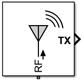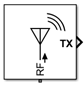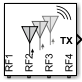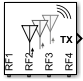# Antenna

Model antenna or antenna array accounting for incident power wave (RX) and radiated power wave (TX)

• Library:
• RF Blockset / Circuit Envelope / Elements

•## Description

Model an antenna or antenna array using the Antenna block. Use this block to:

• Convert a Simulink® input of an incident power wave vector into RF Blockset™ voltage signal at the antenna or antenna array ports.

• Convert current at RF Blockset antenna or antenna array ports to a Simulink output of a radiated power wave vector.

• Introduce antenna impedance into an RF system.

By default, the antenna block is an isotropic radiator producing a Simulink output signal. For an isotropic radiator, specify the gain and impedance of the antenna in the block parameters. The Radiated carrier frequencies parameter is a set of carrier frequencies over-which the Antenna block creates the radiated power wave. For more information, see Radiated Wave and Incident Wave.

The Antenna block mask icons are dynamic: The icons show the current state of the noise parameter, indicate if you have specified an `antennaarray` object in the Antenna object parameter, and update the number of ports based on the number of elements in the `antennaarray` object. This table shows how the block icons vary based on the state of the Simulate noise and Antenna object parameters.

Antenna objectSimulate noise: `on`Simulate noise: `off`
Antenna Catalog ObjectsAntenna Array Catalog Objects## Ports

### Input

expand all

Received signal, specified as a scalar, vector, matrix, or an array of size m-by-n-by-k. In the array:

• m — Represents the frame size

• n — Represents the number of interferers

• k — Represents horizontal or vertical polarization. The value of the k must be 2.

Data Types: `double`

### Output

expand all

Transmitted signal, specified as a scalar, vector, matrix, or an array of size m-by-n-by-k. IN the array:

• m — Represents the frame size

• n — Represents the number of interferers

• k — Represents horizontal or vertical polarization. The value of the k must be 2.

Data Types: `double`

## Parameters

expand all

### Main

Antenna model, specified as one of the following:

• `Isotropic radiator`

• `Antenna Designer`

• `Antenna object`

Note

To use `Antenna Designer` and ```Antenna object``` options you will need Antenna Toolbox™.

Open the Antenna Designer app from the Antenna Toolbox to create an antenna.

#### Dependencies

To enable this parameter, set the Source of antenna model to `Antenna Designer`.

Antenna or array element input from the workspace, specified as a single port antenna element or up to 65 port antenna array elements, or `pcbStack` (Antenna Toolbox) object created using the Antenna Toolbox. Analyze the antenna, array, or PCB stack objects in the workspace for at least one frequency before using them in the block.

For a complete list of the type of antenna and array objects that you can use in this block, see Antenna Catalog (Antenna Toolbox) and Array Catalog (Antenna Toolbox).

#### Dependencies

To enable this parameter, set Source of antenna model to `Antenna object`.

Antenna gain, specified as real scalar or vector if you set units to `dBi` or positive scalar or vector if you set units are `None`. If the antenna gain is a vector, the vector length must be equal to the vector length of Incident carrier frequencies and Radiated carrier frequencies.

#### Dependencies

To enable this parameter, set Source of antenna model to `Isotropic radiator` and check Input incident wave or Output radiated wave or both

Input impedance, specified as a complex-valued scalar or vector in ohms. If the impedance is a vector, the vector length must be equal to the length of Incident carrier frequencies and Radiated carrier frequencies.

#### Dependencies

To enable this parameter, set Source of antenna model to `Isotropic radiator`.

Data Types: `double`
Complex Number Support: Yes

Select this parameter if you want to simulate a receiving antenna.

Select this parameter if you want a simulate a transmitting antenna.

Carrier frequencies for a receiving signal, specified as a nonnegative scalar in hertz or a row vector with each element unit in hertz. If the value of Antenna gain or Impedance is a vector, then the values of Incident carrier frequencies and Radiated carrier frequencies must be identical.

#### Dependencies

To enable this parameter, select .

Carrier frequencies for a transmitting signal, specified as a nonnegative scalar in hertz or a row vector with each element unit in hertz. If the value of Antenna gain or Impedance is a vector, then the values of Incident carrier frequencies and Radiated carrier frequencies must be identical.

#### Dependencies

To enable this parameter, select .

Azimuth and elevation angles towards which the output signal power wave radiates, specified as a finite real row vector of length two with each element unit in degrees or radians.

#### Dependencies

To enable this parameter, set Source of antenna model to `Antenna Designer` or ```Antenna object``` and select .

Azimuth and elevation angles towards which the input signal power wave arrives, specified as a finite real row vector of length two with each element unit in degrees or radians.

#### Dependencies

To enable this parameter, set Source of antenna model to `Antenna Designer` or ```Antenna object``` and select .

Select this parameter to simulate thermal noise in the antenna due to the real part of the impedance see at the antenna terminals. You must select Simulate noise in the Configuration block also.

Select this option to ground and hide the negative terminals. Clear this parameter to expose the negative terminals. By exposing these terminals, you can connect them to other parts of your model.

By default, this option is selected.

### Modeling

Model frequency-dependent antenna parameters, specified as:

• `Time-domain (rationalfit)` — This technique creates an analytical rational model that approximates the whole range of the data.

• `Frequency-domain` — This technique computes the baseband impulse response for each carrier frequency independently. This technique is based on convolution. There is an option to specify the duration of the impulse response. For more information, see Compare Time and Frequency Domain Simulation Options for S-parameters.

The frequency-dependent parameters are:

• Antenna impedance — The input impedance at the antenna terminals. This is used in RF system simulation.

• Normalized vector effective length — A property used that ties between the current flowing at the antenna terminals and the radiated far-field at a given direction. Due to reciprocity, the effective length also ties between the incident field and the induced open-circuit voltage on the antenna terminals.

#### Dependencies

To set source Source of antenna model of `Antenna Designer` or ```Antenna object``` to activate the Modeling Tab that contains the Modeling options parameters.

Relative error acceptable for the rational fit, specified as a scalar. Applies to time-domain modeling of both antenna impedance and normalized vector effective length. The corresponding rational fitting results for each property are displayed on the block mask.

#### Dependencies

To set Modeling options to ```Time domain (rationalfit)``` in .

Select this parameter to automatically calculate the impulse response duration. Clear this parameter to manually specify the impulse response duration using Impulse response duration. Applies to frequency-domain modeling of both antenna impedance and normalized vector effective length.

#### Dependencies

To set this parameter, select `Frequency domain` in Modeling options.

Impulse response duration, specified as a scalar. Applies to frequency-domain modeling of both antenna impedance and normalized vector effective length.

#### Dependencies

To set this parameter, first select `Frequency domain` in Modeling options. Then, clear ```Automatically estimate impulse response duration```.